### IF Function; Understanding It And Different Ways To Use It

The IF function in Excel use different arguments and then return with a value. There is a value that return when the user’s specified terms are evaluated to be true, and another value will return, when the condition is false.

Parameter and Arguments

This is how the IF function’s formula looks like:

IF(logical test,value if true,value if false)

Logical test: This is required. The test that a user wants the function to perform.

Value if true: This is optional. It would be the value that a user wants to return, when/if the logical test argument is true. If it is omitted, it would return with

“true”.

Value if false: This is option. It is the value that should return, if a logical test is false. If omitted, “false” would return.

Example 1: Simple IF Formula

This sample is about acknowledging the information that would help the comprehension of a simple IF formula. In this case, we want to know the amount we make from selling a specific product.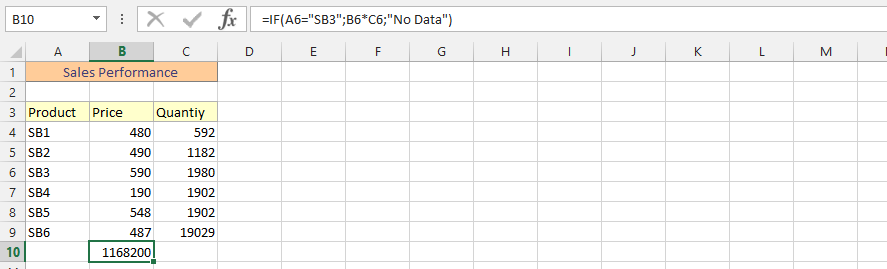Example 2: IF and Average

This is an example that simultaneously uses both the average and if formula.Example 3: IF and SUM

Usage The issue here is that we would like to know how much the company would make, at a level that addresses the total amount that the company would make, in combination with the quantity of products that we’d have in the inventory.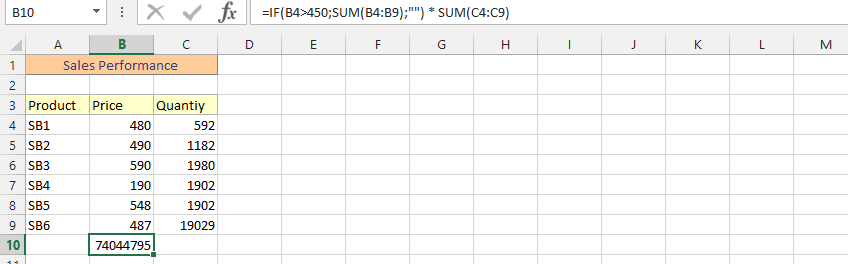Example 4: Double IF in one Formula

The formula here is about using two IF formulas together.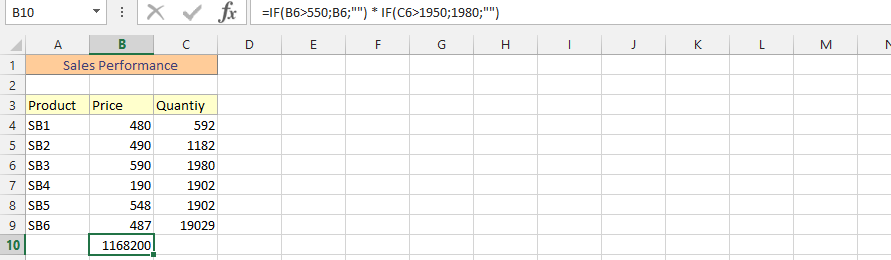Example 5: IF, AVERAGE and SUM

in one Formula This example focuses on combining 3 different formulas into 1 to find a result.Example 6: IF, MIN and MAX

This example use IF, MIN and MAX formulas to find the answer.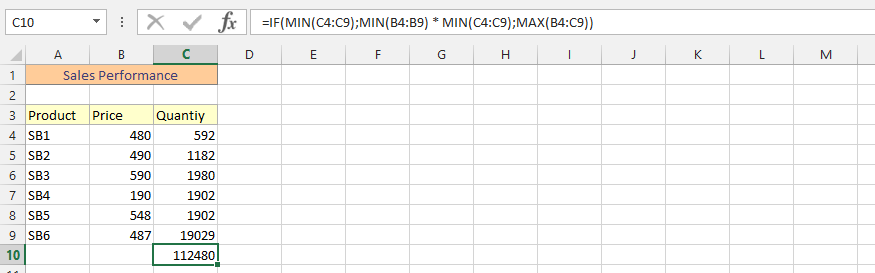Example 7: IF and Date

This is the usage of IF and Date formula. This sample focuses on how much we’ve made today, if we only focus on a specific price.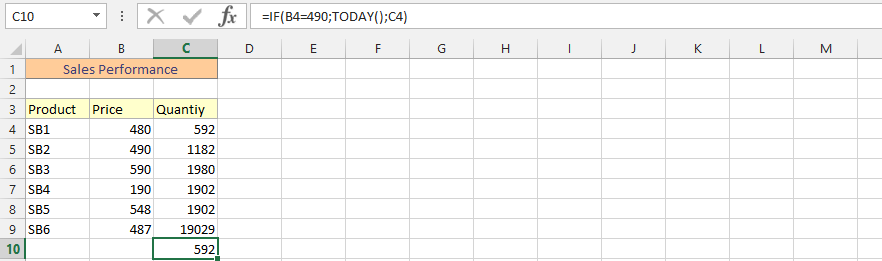Example 8: Double IF in one Formula

This example use two IF formula, because we would like to start a new project that would allow us to cleanse our remaining inventory. It was intended that in the event that we have not sold all the products after a specific period of time, we were going to sell it for a specific amount of money. Usage of double IF formula allow productivity to help determine how much we would make from selling it in a specific project.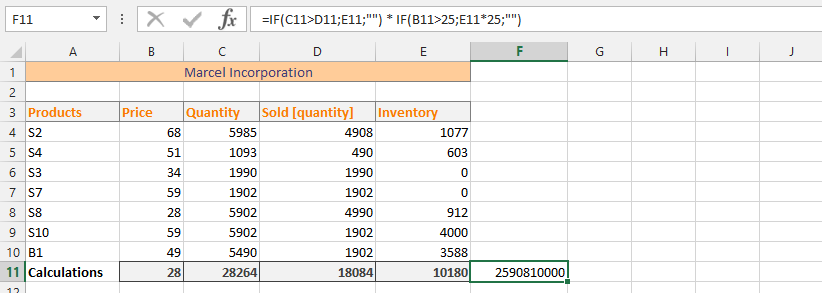Example 9: Net Worth after Expenses

We want to know the profit we would make after paying all expenses, and selling the products at a specific price. This is where we would be using double IF formulas and SUM.Example 10: SUM and IF

This is the usage of both functions: sum and if – and find the answer that we are looking for.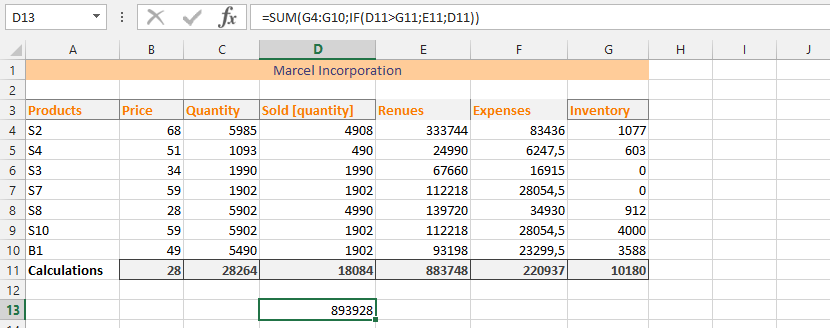## Template

```Further reading: